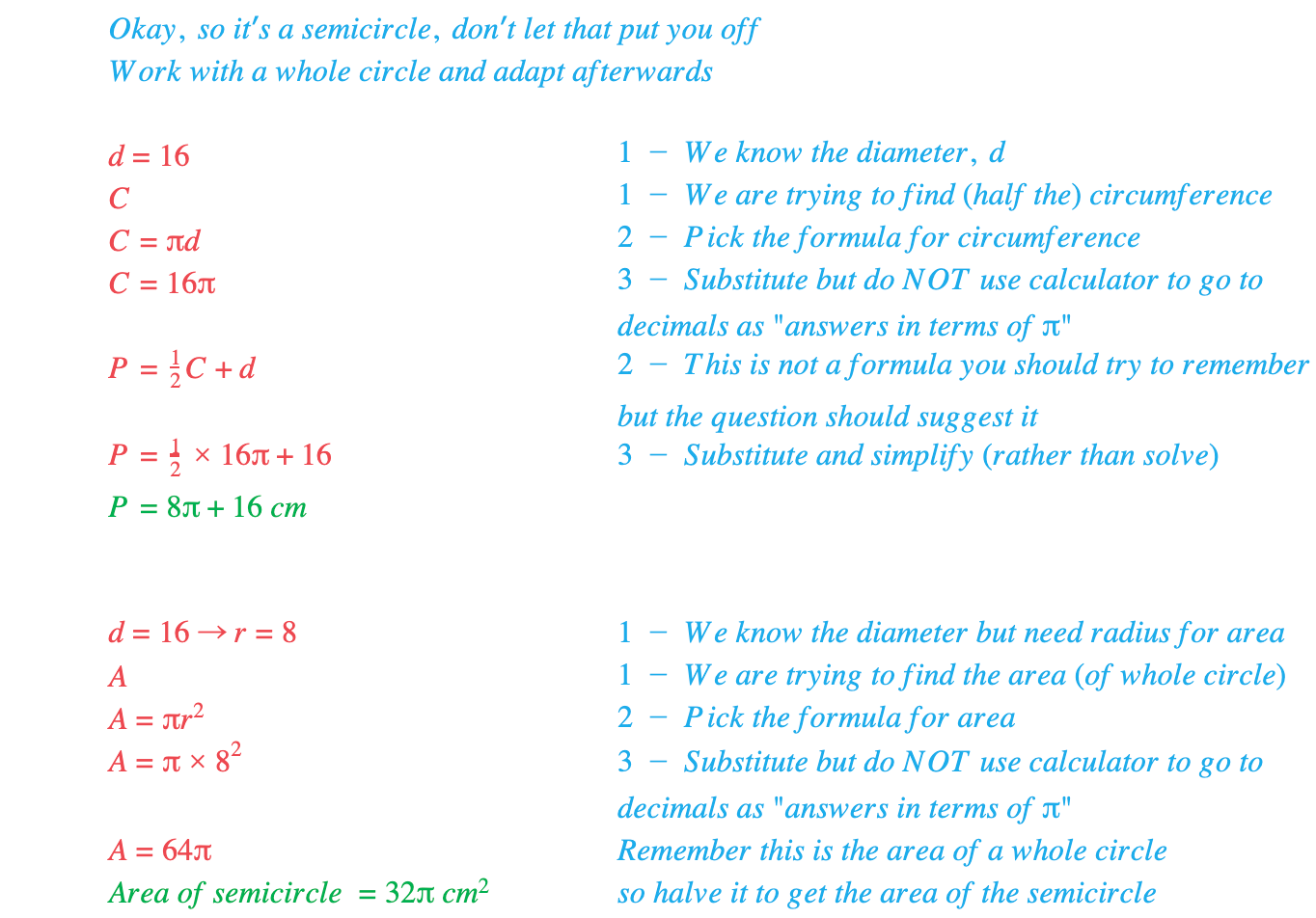# Edexcel IGCSE Maths 复习笔记 4.12.1 Circles - Area & Circumference

Edexcel IGCSE Maths 复习笔记 4.12.1 Circles - Area & Circumference

#### Why are circles different to other 2D shapes?

• Circles just have their own language!
• The circumference of a circle is its perimeter
• π is the number (3.14159 …) that links a circle’s diameter to its circumference
• Diameter (d) is twice the radius (r)
• You may be asked to give an area answer to a certain number of decimal places or significant figures
• Alternatively you may be asked to give the exact value – or “give your answer in terms of π” – so this topic could crop up on the non-calculator paper!

#### Working with circles

• You must know the formulas for the area and circumference of a circle
• There are two versions for the circumference and it is important not to get the radius and diameter confused
• Remember that d = 2rBut you may prefer to remember the formulas by having different letters involved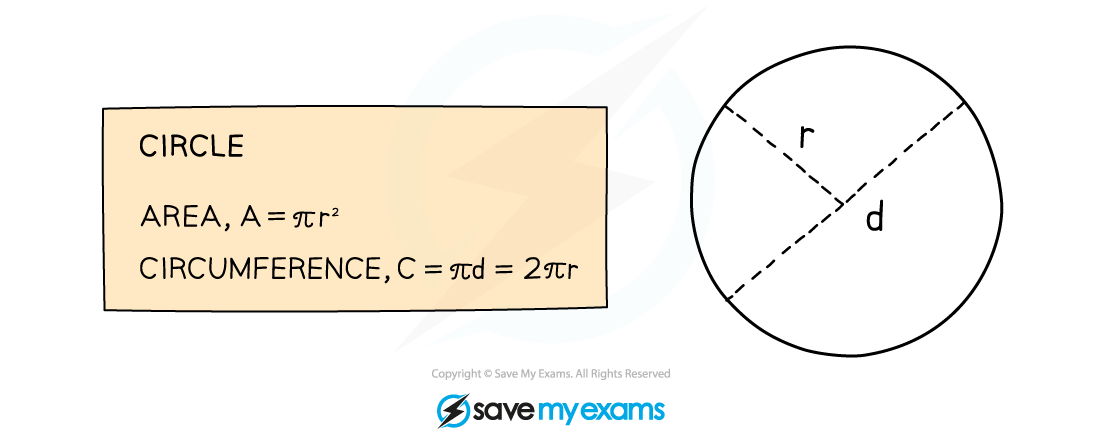• Other than that, working with circle formulas is just like working with any other formula:
1. WRITE DOWN – what you know (what you want to know)
2. Pick correct FORMULA
3. SUBSTITUTE and SOLVE

#### Worked Example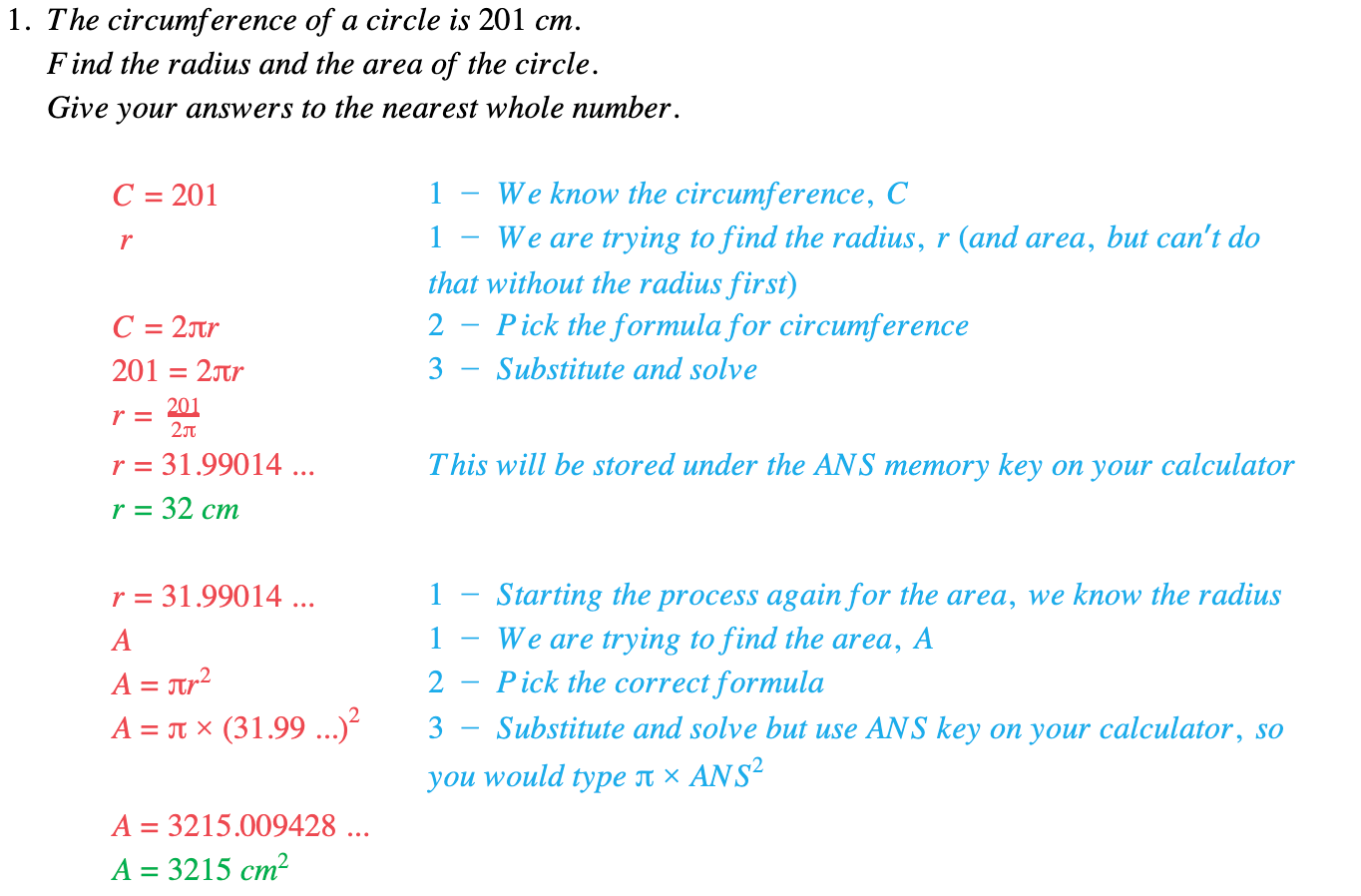#### Worked Example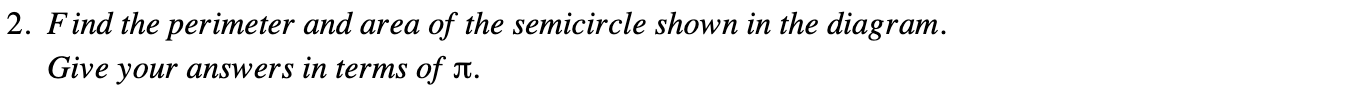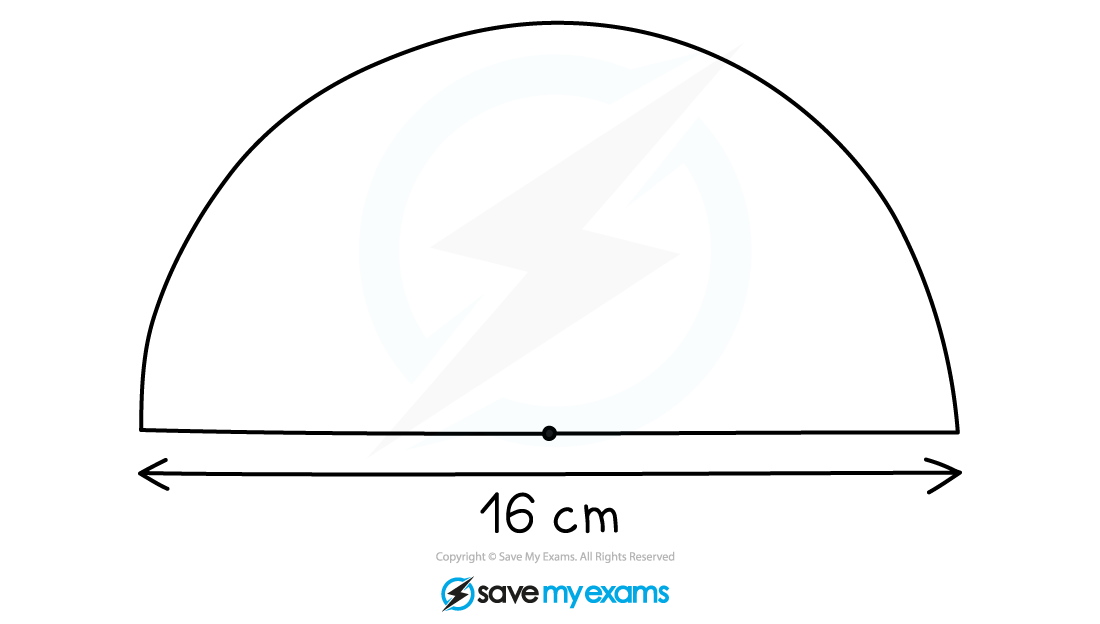#### Worked Example